# Rhombicosidodecahedron

Rhombicosidodecahedron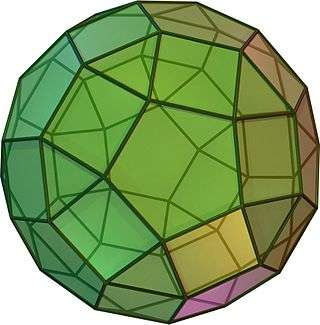TypeArchimedean solid
Uniform polyhedron
ElementsF = 62, E = 120, V = 60 (χ = 2)
Faces by sides20{3}+30{4}+12{5}
Schläfli symbolsrr{5,3} ort0,2{5,3}
Wythoff symbol3 5 | 2
Coxeter diagramSymmetry groupIh, H3, [5,3], (*532), order 120
Rotation groupI, [5,3]+, (532), order 60
Dihedral Angle3-4: 159°05′41″ (159.09°)
4-5: 148°16′57″ (148.28°)
ReferencesU27, C30, W14
PropertiesSemiregular convex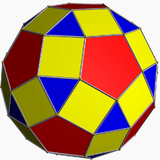Colored faces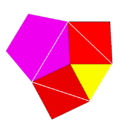3.4.5.4
(Vertex figure)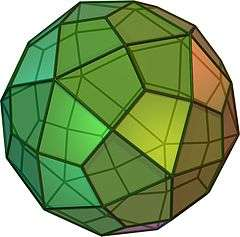Deltoidal hexecontahedron
(dual polyhedron)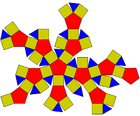Net

In geometry, the rhombicosidodecahedron, or small rhombicosidodecahedron, is an Archimedean solid, one of thirteen convex isogonal nonprismatic solids constructed of two or more types of regular polygon faces.

It has 20 regular triangular faces, 30 square faces, 12 regular pentagonal faces, 60 vertices and 120 edges.

The name rhombicosidodecahedron refers to the fact that the 30 square faces lie in the same planes as the 30 faces of the rhombic triacontahedron which is dual to the icosidodecahedron.

It can also be called an expanded or cantellated dodecahedron or icosahedron, from truncation operations on either uniform polyhedron.

## Geometric relations

If you expand an icosahedron by moving the faces away from the origin the right amount, without changing the orientation or size of the faces, and do the same to its dual dodecahedron, and patch the square holes in the result, you get a rhombicosidodecahedron. Therefore, it has the same number of triangles as an icosahedron and the same number of pentagons as a dodecahedron, with a square for each edge of either.

The rhombicosidodecahedron shares the vertex arrangement with the small stellated truncated dodecahedron, and with the uniform compounds of six or twelve pentagrammic prisms.

The Zometool kits for making geodesic domes and other polyhedra use slotted balls as connectors. The balls are "expanded" rhombicosidodecahedra, with the squares replaced by rectangles. The expansion is chosen so that the resulting rectangles are golden rectangles.

Twelve of the 92 Johnson solids are derived from the rhombicosidodecahedron, four of them by rotation of one or more pentagonal cupolae: the gyrate, parabigyrate, metabigyrate and trigyrate rhombicosidodecahedron. Eight more can be constructed by removing up to three cupolae, sometimes also rotating one or more of the other cupolae.

## Cartesian coordinates

Cartesian coordinates for the vertices of a rhombicosidodecahedron with edge length 2 centered at the origin are all even permutations of:

(±1, ±1, ±φ3),
(±φ2, ±φ, ±2φ),
(±(2+φ), 0, ±φ2),

where φ = 1 + 5/2 is the golden ratio.

## Orthogonal projections

The rhombicosidodecahedron has five special orthogonal projections, centered, on a vertex, on two types of edges, and three types of faces: triangles, squares, and pentagons. The last two correspond to the A2 and H2 Coxeter planes.

Orthogonal projections
Centered by Vertex Edge
3-4
Edge
5-4
Face
Square
Face
Triangle
Face
Pentagon
Image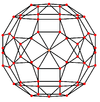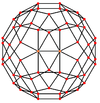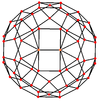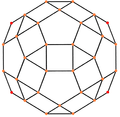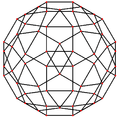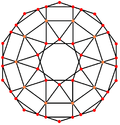Projective
symmetry
     
Dual
image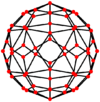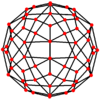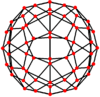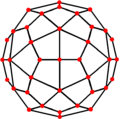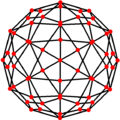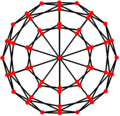## Spherical tiling

The rhombicosidodecahedron can also be represented as a spherical tiling, and projected onto the plane via a stereographic projection. This projection is conformal, preserving angles but not areas or lengths. Straight lines on the sphere are projected as circular arcs on the plane.

 Orthographic projection Stereographic projections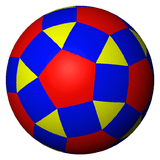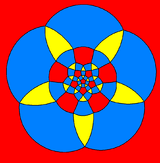Pentagon-centered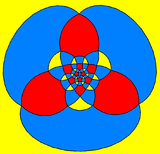Triangle-centered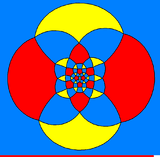Square-centered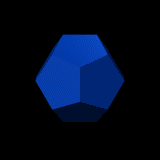Expansion of either a dodecahedron or an icosahedron creates a rhombicosidodecahedron.

### Symmetry mutations

This polyhedron is topologically related as a part of a sequence of cantellated polyhedra with vertex figure (3.4.n.4), which continues as tilings of the hyperbolic plane. These vertex-transitive figures have (*n32) reflectional symmetry.

### Johnson solids

There are 13 related Johnson solids, 5 by diminishment, and 8 including gyrations:

 J5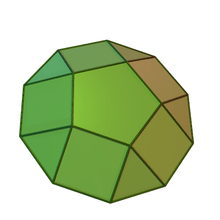76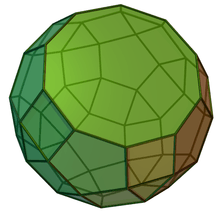80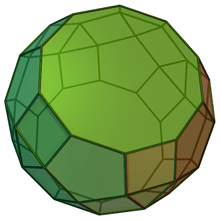81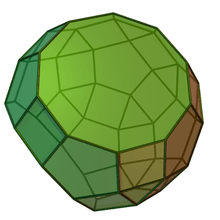83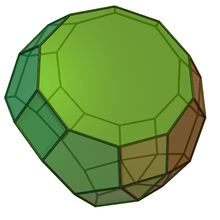72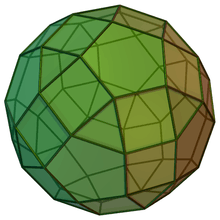73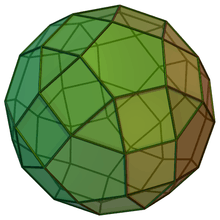74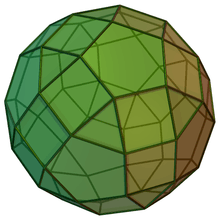75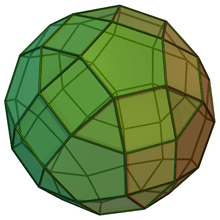77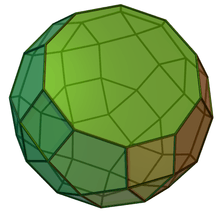78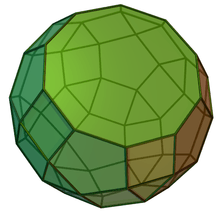79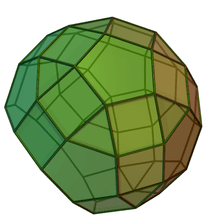82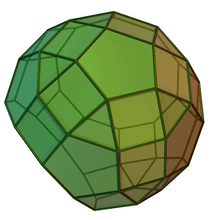### Vertex arrangement

The rhombicosidodecahedron shares its vertex arrangement with three nonconvex uniform polyhedra: the small stellated truncated dodecahedron, the small dodecicosidodecahedron (having the triangular and pentagonal faces in common), and the small rhombidodecahedron (having the square faces in common).

It also shares its vertex arrangement with the uniform compounds of six or twelve pentagrammic prisms.Rhombicosidodecahedron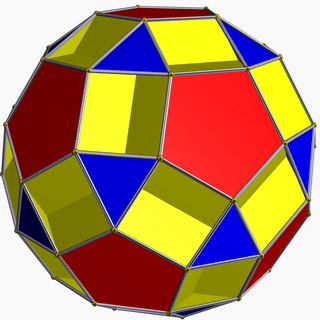Small dodecicosidodecahedron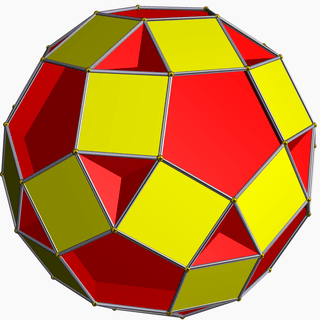Small rhombidodecahedron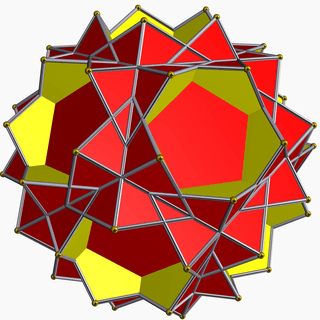Small stellated truncated dodecahedron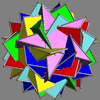Compound of six pentagrammic prisms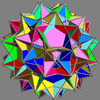Compound of twelve pentagrammic prisms

## Rhombicosidodecahedral graph

Rhombicosidodecahedral graph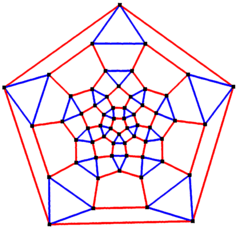5-fold symmetry
Vertices 60
Edges 120
Automorphisms 120
Properties Quartic graph, Hamiltonian, regular

In the mathematical field of graph theory, a rhombicosidodecahedral graph is the graph of vertices and edges of the rhombicosidodecahedron, one of the Archimedean solids. It has 60 vertices and 120 edges, and is a quartic graph Archimedean graph.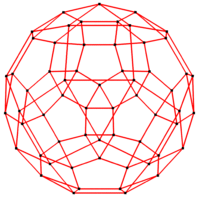3-fold symmetry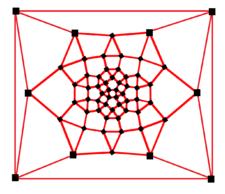4-fold symmetry